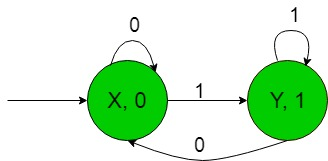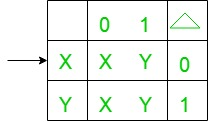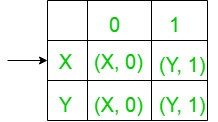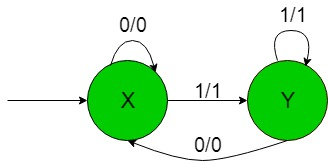# Conversion of Moore to Mealy machine (Set 9)

• Difficulty Level : Easy
• Last Updated : 21 Jan, 2022

Prerequisite: Mealy and Moore Machines, Difference between Mealy machine and Moore machine
In this article, we shall see a conversion of Moore to Mealy machine-

State Transition Diagram of a Moore machine:-Above Moore machine takes the binary number {0, 1} as input and produce residue modulo ‘2’ as output i.e, when the equivalent decimal number of binary input over {0, 1} is divided by 2 then it gives output as it’s remainder.
Now we need to transform the above transition diagram of Moore machine to equivalent Mealy machine transition diagram.
Steps for the required conversion are given below:-

• Step-1: Formation of State Transition Table of the above Moore machine-• In the above transition table, States ‘X’ and ‘Y’ are kept in the first column which on getting ‘0’ as the input it transits to ‘X’ and ‘X’ states respectively, kept in the second column and on getting ‘1’ as the input it transits to ‘Y’ and ‘Y’ states respectively, kept in the third column and In the fourth column under Δ, there are corresponding outputs of the first column states. In the table, An arrow (→) indicates the initial state.

• Step-2: Formation of Transition Table for Mealy machine from above Transition Table of Moore machine-
Below transition table is going to be formed with the help of the above table and its entries just by using the corresponding output of the states of the first column and placing them in the second and third column accordingly.• In the above table, the states in the first column like ‘X’ on getting ‘0’ as the input it goes to a state ‘X’ and gives ‘0’ as the output and on getting ‘1’ as the input it goes to the state ‘Y’ and gives ‘1’ as the output and so on for the remaining states in the first column. In the table, An arrow (→) indicates the initial state.

• Step-3: Then finally we can form the state transition diagram of Mealy machine with help of it’s above transition table.
The required diagram is shown below-• Above Mealy machine takes the binary number {0, 1} as input and produce residue modulo ‘2’ as output i.e, when the equivalent decimal number of binary input over {0, 1} is divided by 2 then it gives output as it’s remainder.

Note: While converting from Moore to Mealy machine the number of states remains same for both Moore and Mealy machine but in case of Mealy to Moore conversion it does not give the same number of states.

My Personal Notes arrow_drop_up